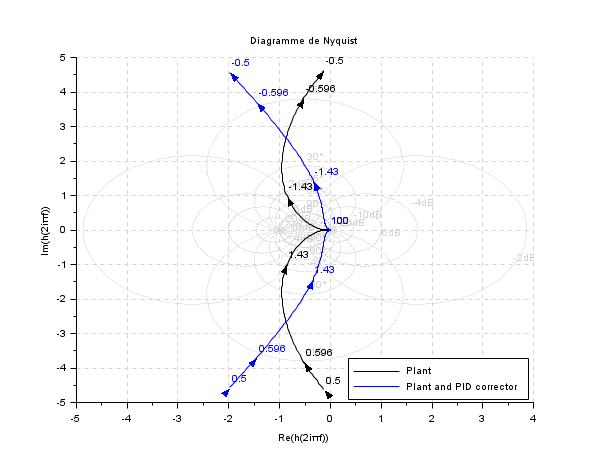Scilab Home page | Wiki | Bug tracker | Forge | Mailing list archives | ATOMS | File exchange
Change language to: English - Português - 日本語 - Русский

# hallchart

Draws the Hall chart

### Syntax

`hallchart([ modules [,args [,colors]]])`

### Arguments

modules

real vector ( modules (in dB))

args

real vector (phases (in degree))

colors

a scalar or a vector, the color indices for isogain and iso phase curves

### Description

plot the Hall'chart: iso-module and iso-argument contours of `y/(1+y)` in the `real(y)`, `imag(y)` plane

`hallchart` may be used in conjunction with nyquist.

The default values for `modules` and `args` are respectively :

`[-20 -10 -6 -4 -2 2 4 6 10 20]`

`[-90 -60 -45 -30 -15 15 30 45 60 90]`

### Graphics entities organization

The `hallchart` function create a single compound object which is generally the last child of the current axes. This compound object contains a set of compound objects, one for each grid curve. The first ones are the iso module curves and the last one the iso-argument contours. Each of these compound objects contains a Polyline object (the curve) and a Text object (the label). The following piece of code can be used to change the color of the ith iso module curve:

```clf();hallchart()
ax=gca();//handle on current axes
c=ax.children(\$).children;// the handles on the chart grid curves
i=4; //the index of the -4dB curve
ci=c(i); //the handle on the -4dB curve
ci.children(1).foreground=color('red'); //draw it in red

j=3; // the index of the -45° curve
cj=c(10+j); //the handle on the -45° curve
cj.children(1).thickness=3;//draw it thicker```### Examples

```//Hall chart
clf();hallchart()``````//Hall chart as a grid for nyquist
s=poly(0,'s');
Plant=syslin('c',16000/((s+1)*(s+10)*(s+100)));
//two degree of freedom PID
tau=0.2;xsi=1.2;
PID=syslin('c',(1/(2*xsi*tau*s))*(1+2*xsi*tau*s+tau^2*s^2));
clf();
nyquist([Plant;Plant*PID],0.5,100,["Plant";"Plant and PID corrector"]);
hallchart(colors=color('light gray')*[1 1])
//move the caption in the lower right corner
ax=gca();Leg=ax.children(1);
Leg.legend_location="in_lower_right";```